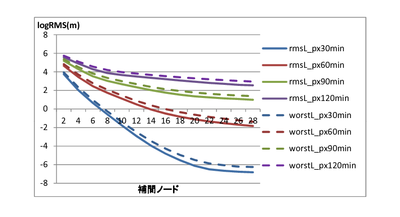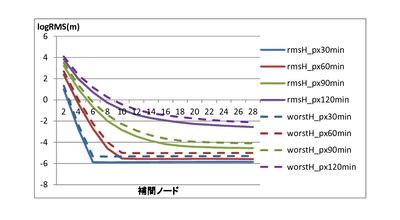# The optimal interpolation for the orbit of satellites

Graduation Report by Osamu Muto

We compare three interpolations – Lagrange interpolation, Hermete interpolation and third order Spline interpolation – using the orbital data of four satellites – GPS-36, LAGEOS-1, Ajisai, and Envisat. We calculate optimal pair of interpolation nodes and data interval which satisfy conditions that both RMS of errors and the worst error are smaller than 1 mm in position and 1 micron/s in velocity. We find that Hermite interpolation is more desirable than Lagrange interpolation and third order Spline interpolation as both points of interpolation nodes and distance between data. However, the results imply that we can interpolate with Lagrange interpolation in lower order when we use short interval data.Lagrange interpolation case.  Along-track error of GPS-36 orbits.Hermite interpolation case.  Along-track error of GPS-36 orbit

s.